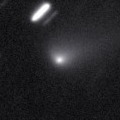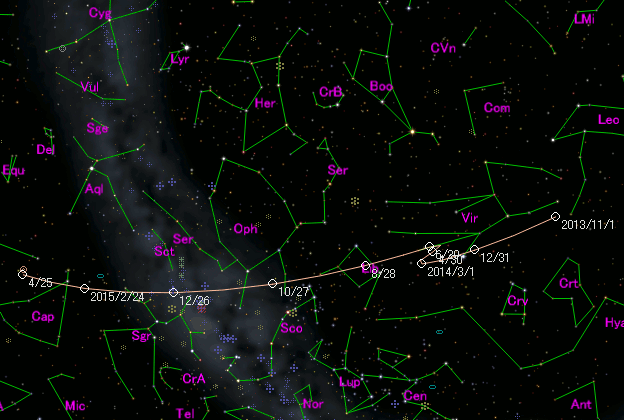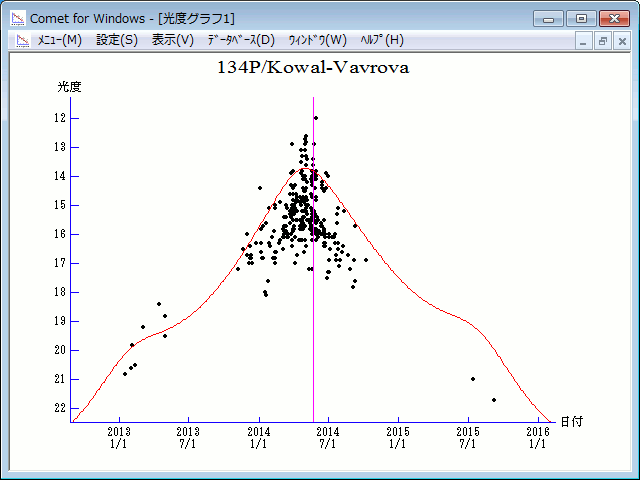\$B%3%o%k(B-\$B%t%!!<%t%m%t%!!

134P/Kowal-Vavrova (2014)\$B\$B50F;MWAG(B

```Epoch 2014 May 23.0 TT = JDT 2456800.5
T 2014 May 21.49482 TT                                  MPC
q   2.5713321            (2000.0)            P               Q
n   0.06336331     Peri.   18.58418     -0.75842496     +0.65113461
a   6.2312828      Node   202.12064     -0.60717999     -0.72179458
e   0.5873511      Incl.    4.34878     -0.23690514     -0.23459817
P  15.6
From 902 observations 1983 May 8-2014 July 3, mean residual 0".6.
Nongravitational parameters A1 = +3.52, A2 = +0.1405.
```\$B@1?^(B\$B8wEYJQ2=(B

```        m1 = 4.5 + 5 log\$B&\$(B + 20.0 log r
```\$B50F;MWAG\$O(BM.P.E.C. 2014-N57\$B\$K7G:\\$5\$l\$?\$b\$N\$G\$9!#(B \$B@1?^\$O%9%F%i%J%S%2!<%?(B Ver.8 (\$B%"%9%H%m%"!<%D(B) \$B\$G:n@.\$7\$?\$b\$N\$G\$9!#(B \$B8wEY%0%i%U\$O(BComet for Windows\$B\$G:n@.\$7\$?\$b\$N\$G\$9!#(B